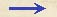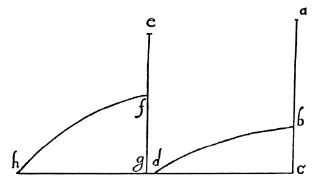Discorsi Propositions 3/09-th-06 Discorsi Proposition3/09-th-06THEOREMA, PROPOSITIO IX. THEOREM. PROPOSITION IXAequalis sunt amplitudines parabolarum, quarum altitudines et sublimitates e contrario sibi respondent. The amplitudes of two parabolas are equal when their altitudes and sublimities are inversely proportional. Parabolae fh altitudo gf ad altitudinem cb parabolae bd eandem habeat rationem, quam sublimitas ba ad sublimitatem fe: dico, amplitudinem hg amplitudini dc esse aequalem.Cum enim prima gf ad {10} secundam cb eandem habeat rationem quam tertia ba ad quartam fe, rectangulum gfe, primae et quartae, aequale erit rectangulo cab, secundae et tertiae; ergo quadrata quae hisce rectangulis aequalia sunt, aequalia erunt inter se: rectangulo vero gfe aequale est quadratum dimidiae gh; rectangulo autem cba aequale est quadratum dimidiae cd: ergo quadrata haec, et eorum latera, et laterum dupla, aequalia erunt.Haec {20} autem sunt amplitudines gh, cd: ergo patet propositum. Let the altitude gf of the parabola fh bear to the altitude cb of the parabola bd the same ratio which the sublimity ba bears to the sublimity fe; then I say the amplitude hg is equal to the amplitude dc. For since the first of these quantities, gf, bears to the second cb the same ratio which the third, ba, bears to the fourth fe, it follows that the area of the rectangle gf.fe is equal to that of the rectangle cb.ba; therefore squares which are equal to these rectangles are equal to each other. (Condition 3/05-pr-02-cor) But [by Proposition VI] the square of half of gh is equal to the rectangle gf.fe; and the square of half of cd is equal to the rectangle cb.ba. Therefore these squares and their sides and the doubles of their sides are equal. But these last are the amplitudes gh and cd. Hence follows the proposition.Discorsi Propositions 3/09-th-06 Discorsi Proposition3/09-th-06